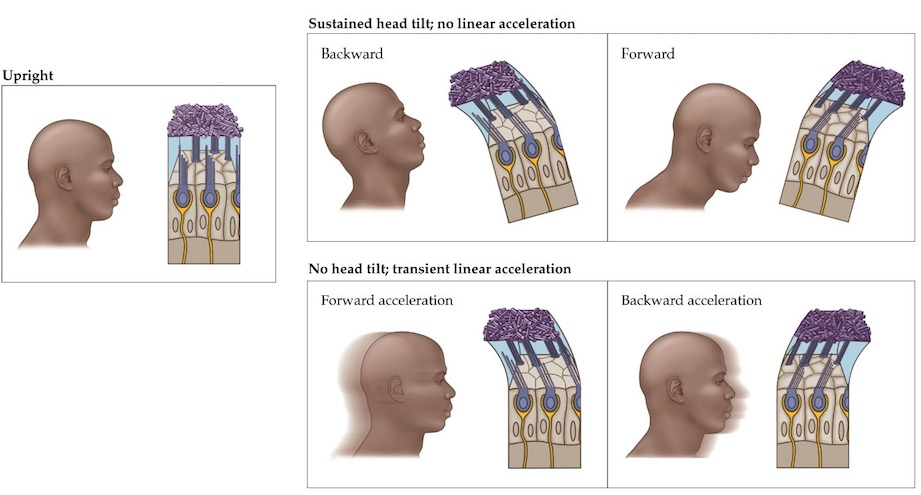# Sensation & Perception 5e Web Essay 12.1 - Gravity versus Linear Acceleration

For convenience, we sometimes conceptualize linear acceleration as a force (e.g., centrifugal force). Other times we think of the force of gravity as being created by an equivalent upward linear acceleration. But equivalence does not prove that gravity and linear acceleration are the same thing; some physicists, though, have hypothesized this to be the case.

Let’s illustrate the classical difference between gravity and linear acceleration using a simple example. Sit down. You are experiencing a gravitational force that is keeping you from floating off of your chair, right? You are relatively stationary and not accelerating, right? Hence, you are simultaneously experiencing a gravitational force and not linearly accelerating. Therefore, at least in the classical sense, gravity is not a linear acceleration.

However, gravity can cause a linear acceleration. For example, if you fall from the seat of your chair, you will be linearly accelerated by gravity until you hit the ground. In fact, while airborne, you actually will be experiencing weightlessness as you linearly accelerate toward the floor. Pardon the pun, but all these physics may be enough to make your head spin. If so, the important things to remember are: 1) gravity and linear acceleration are equivalent; and 2) the equivalence of gravity and linear acceleration leads to an ambiguous signal being provided to the brain by the otolith organs (see figure below).Forces acting on the head result in displacement of the otoconia. This example illustrates displacement of the utricular macula. For each of the head tilts and linear accelerations, some set of hair cells will be maximally excited, whereas another set will be maximally inhibited, according to the orientation of the hair cells. Note that head tilts and linear accelerations—when matched in direction and magnitude—produce similar otoconial movement, demonstrating that the otolith organs respond to both gravity and linear acceleration.